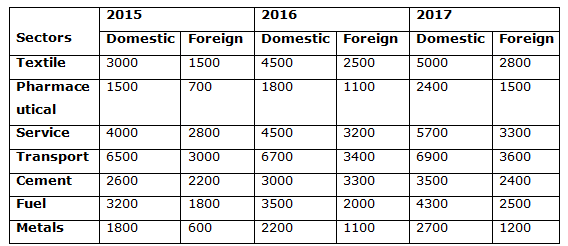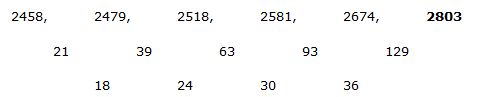# IBPS PO Prelims 2018 – Quantitative Aptitude Questions Day-3

Dear Readers, Bank Exam Race for the Year 2018 is already started, To enrich your preparation here we have providing new series of Practice Questions on Quantitative Aptitude – Section. Candidates those who are preparing for IBPS PO Prelims 2018 Exams can practice these questions daily and make your preparation effective.

IBPS PO Prelims 2018 – Quantitative Aptitude Questions Day – 3

Directions (Q. 1 – 5) Study the following information carefully and answer the given questions:

The following table shows the total investment (In Crores) in various sectors in different years.1) Find the difference between the total investments in textile sector to that of total investment in Cement sector in all the given years together?

a) 3200

b) 2300

c) 2000

d) 1700

e) None of these

2) Find the ratio between the total domestic investments in Transport to that of total domestic investment in Fuel in all the given years together?

a) 157: 92

b) 52: 17

c) 11: 5

d) 201: 110

e) None of these

3) Find the average number of domestic investment in the year 2015 in all the given sectors together except service and metal sectors?

a) 3360

b) 3200

c) 2870

d) 2950

e) None of these

4) Total foreign investment of Transport and Fuel sector in the year 2016 is approximately what percentage of total domestic investment of Pharmaceuticals and cement sector in the year 2017?

a) 108 %

b) 84 %

c) 92 %

d) 66 %

e) 75 %

5) Total domestic investment in all the given sectors in the year 2017 is approximately what percentage more than the total foreign investment in all the given sectors in the year 2017?

a) 85 %

b) 92 %

c) 58 %

d) 64 %

e) 76 %

Directions (Q. 6 – 10): What value should come in place of question mark (?) in the following number series?

6) 56, 57, 112, 339, 1352, ?

a) 5178

b) 4357

c) 6765

d) 3329

e) 2593

7) 51, 26, 27, 42, 86, 217.5, ?

a) 655.5

b) 587.5

c) 442.5

d) 719.5

e) 838.5

8) 13, 17, 67, 243, 1251, ?

a) 5213

b) 3178

c) 2356

d) 7457

e) 6592

9) 21, 29, 85, 319, 1401, ?

a) 5274

b) 7221

c) 6155

d) 6372

e) 4173

10) 2458, 2479, 2518, 2581, 2674, ?

a) 2961

b) 2835

c) 2846

d) 2917

e) 2803

Direction (1-5) :

The total investments in textile sector in all the given years together

= > 3300 + 1500 + 4500 + 2500 + 5000 + 2800 = 19300

The total investments in cement sector in all the given years together

= > 2600 + 2200 + 3000 + 3300 + 3500 + 2400 = 17000

Required difference = 19300 – 17000 = 2300

The total domestic investment in Transport in all the given years together

= > 6500 + 6700 + 6900 = 20100

The total domestic investment in Fuel in all the given years together

= > 3200 + 3500 + 4300 = 11000

Required ratio = 20100: 11000 = 201: 110

The total number of domestic investment in the year 2015 in all the given sectors together except service and metal sectors

= > 3000 + 1500 + 6500 + 2600 + 3200 = 16800

Required average = 16800/5 = 3360

Total foreign investment of Transport and Fuel sector in the year 2016

= > 3400 + 2000 = 5400

Total domestic investment of Pharmaceuticals and cement sector in the year 2017

= > 2400 + 3500 = 5900

Required % = (5400/5900)*100 = 92 %

Total domestic investment in all the given sectors in the year 2017

= > 5000 + 2400 + 5700 + 6900 + 3500 + 4300 + 2700 = 30500

Total foreign investment in all the given sectors in the year 2017

= > 2800 + 1500 + 3300 + 3600 + 2400 + 2500 + 1200 = 17300

Required % = [(30500 – 17300)/17300]*100 = 76 %

Direction (6-10) :

The pattern is, *1 + 1, *2 – 2, *3 + 3, *4 – 4, *5 + 5,..

The pattern is, *0.5 + 0.5, *1 +1, *1.5 +1.5, *2 +2, *2.5 +2.5, *3 + 3,….

Explanation:

The pattern is, *2 – 32, *3 + 42, *4 – 52, *5 + 62, *6 – 72,..

The pattern is, *1 + 23, *2 +33, *3 + 43, *4 + 53, *5 + 63,..The difference of difference is, 18, 24, 30, 36,..